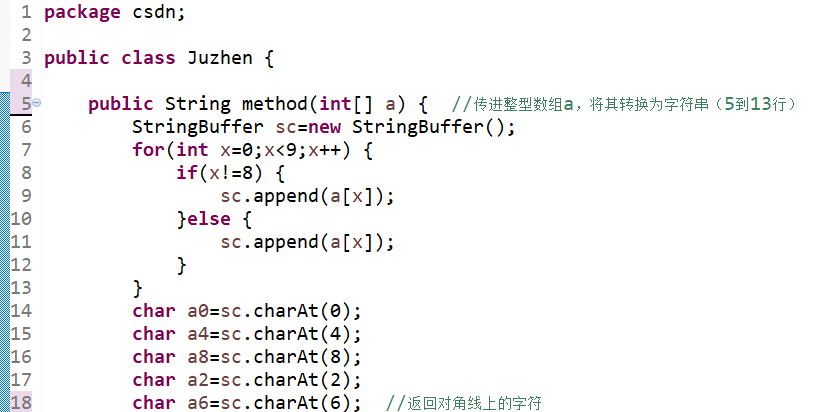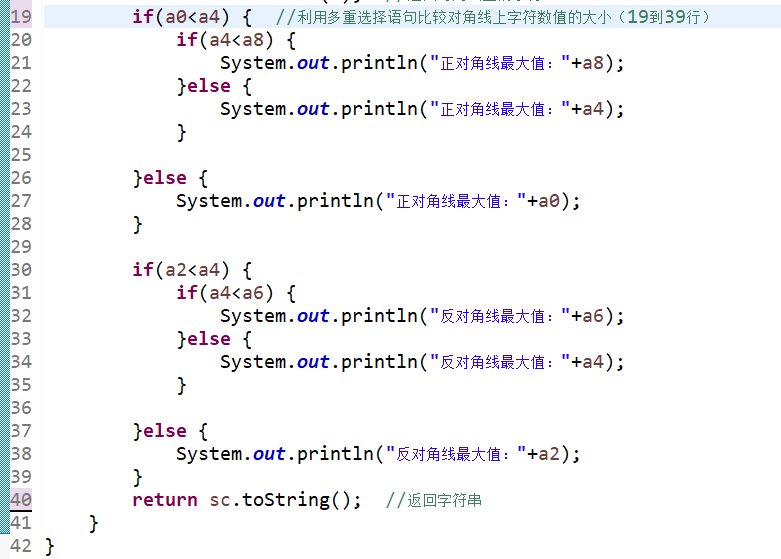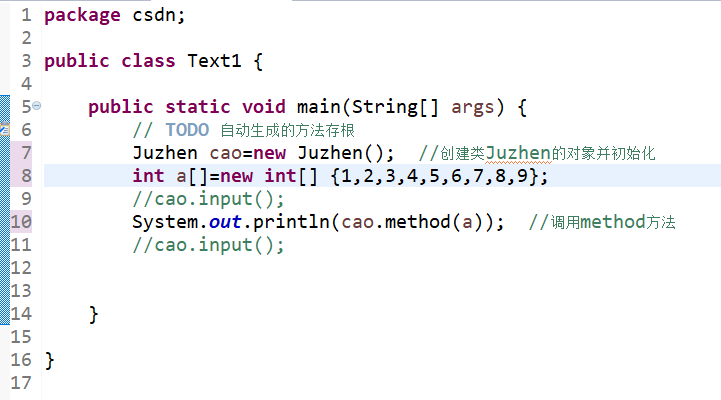2020-05-14 08:30

# 高分悬赏：Java语言编写一个程序，分别计算3x3矩阵的两条对角线上最大的值

• 写回答
• 关注问题
• 收藏
• 邀请回答

#### 3条回答默认 最新

•毕小宝 2020-05-16 18:16
已采纳

实现思路：分析下对角线数据的特点就是，例如 N* N 的数组 a ,对角线 a[i][i] ，遍历数组求最大值就可以了。
编码这件事情，还是自己写一下才能进步呢。

已采纳该答案
打赏 评论
•穆暖阳 2020-05-14 08:40

俩天对角线特点 i+j == 2 i==j 然后符合条件的乘法就ok了 之后比较

`````` #include <stdio.h>
int main()
{
int a;
int i = 0;
int j = 0;
int sum1 = 1;
int sum2 = 1;

for(i = 0 ; i < 3 ; i++ )
for(j = 0 ; j < 3 ; j++)
scanf_s("%d",&a[i][j]);

for(i = 0 ; i < 3 ; i++ )
{
for(j = 0 ; j < 3 ; j++)
{
printf("%d    ",a[i][j]);
}
printf("\n");
}

for(i = 0 ; i < 3 ; i++ )
for(j = 0 ; j < 3 ; j++)
{
if( i==j )
sum1 *= a[i][j];
if( (i+j) == 2)
sum2 *= a[i][j];
}
if(sum1>sum2)
printf("sum1 = %d\n",sum1);
else
printf("sum2 = %d\n",sum2);

getchar();
getchar();
return 0;
``````

}

打赏 评论
•水️ 2020-05-15 08:35

这是用JAVA写的，在第三张图片的初始化中按行输入矩阵对应位置的值。我也是刚学JAVA，弄了三个多小时，马马虎虎弄出结果了（太笨了），代码还有
很多瑕疵，请多多包涵（哈哈哈哈哈）打赏 评论